# JP4400395B2 - Position calculation method and program thereof - Google Patents

## Info

Publication number
JP4400395B2
JP4400395B2 JP2004278656A JP2004278656A JP4400395B2 JP 4400395 B2 JP4400395 B2 JP 4400395B2 JP 2004278656 A JP2004278656 A JP 2004278656A JP 2004278656 A JP2004278656 A JP 2004278656A JP 4400395 B2 JP4400395 B2 JP 4400395B2
Authority
JP
Japan
Prior art keywords
position
distance measurement
measurement result
detection target
error
Prior art date
Legal status (The legal status is an assumption and is not a legal conclusion. Google has not performed a legal analysis and makes no representation as to the accuracy of the status listed.)
Expired - Fee Related
Application number
JP2004278656A
Other languages
Japanese (ja)
Other versions
JP2006090913A (en
Inventor

Original Assignee

Priority date (The priority date is an assumption and is not a legal conclusion. Google has not performed a legal analysis and makes no representation as to the accuracy of the date listed.)
Filing date
Publication date
Application filed by 株式会社日立製作所 filed Critical 株式会社日立製作所
Priority to JP2004278656A priority Critical patent/JP4400395B2/en
Publication of JP2006090913A publication Critical patent/JP2006090913A/en
Application granted granted Critical
Publication of JP4400395B2 publication Critical patent/JP4400395B2/en
Application status is Expired - Fee Related legal-status Critical
Anticipated expiration legal-status Critical

## Images

••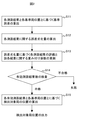•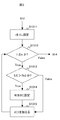•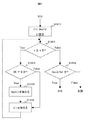•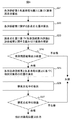•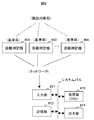•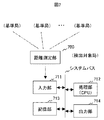## Classifications

• GPHYSICS
• G01MEASURING; TESTING
• G01S5/00Position-fixing by co-ordinating two or more direction or position line determinations; Position-fixing by co-ordinating two or more distance determinations
• G01S5/02Position-fixing by co-ordinating two or more direction or position line determinations; Position-fixing by co-ordinating two or more distance determinations using radio waves
• G01S5/0205Details
• G01S5/021Calibration, monitoring or correction
• HELECTRICITY
• H04ELECTRIC COMMUNICATION TECHNIQUE
• H04WWIRELESS COMMUNICATION NETWORKS
• H04W64/00Locating users or terminals or network equipment for network management purposes, e.g. mobility management

## Description

The present invention relates to a position calculation method, and more particularly to a method for calculating the position of a wireless device based on a result of distance measurement using electromagnetic waves.

For example, according to Non-Patent Document 1, the GPS receiver receives signals from a plurality of GPS satellites, and detects the position of the receiver from each received signal.

According to Patent Document 1, a plurality of radio base stations receive signals from a radio terminal, and the position of the radio terminal is detected from each received signal.

In any of the above techniques, as shown in FIG. 1, a plurality of radio devices (hereinafter referred to as reference stations) 101, 102,..., Whose positions P i (i = 1, 2,..., N) are known. Based on the measurement of the reception time of the radio signal between 10n and the radio device 100 whose position P is unknown (hereinafter referred to as the detection target station) 100, the distance between the reference station and the detection target station is expressed as R i (i = 1, 2, ..., n). Then, P is calculated based on R i (i = 1, 2,..., N) and P i (i = 1, 2,..., N).

Although the above two techniques have the difference that the reception time is measured differently, in principle, the position P is obtained as a solution of simultaneous equations, Equation 3 .

In Equation 3 , || x || is the magnitude of the vector x, c is the speed of the radio wave, and T is a clock used as a reference when measuring the distance R i (i = 1, 2,..., N). Error.

In Equation 3 , the unknowns are P and T. When P is a point on the two-dimensional space, n ≧ 3, and when P is a point on the three-dimensional space, the above solution is obtained when n ≧ 4. Obtainable.
By the way, when such a wireless position detection system is operated in an environment where reflection of a wireless signal can easily occur, such as indoors, waveform distortion of the wireless signal is likely to occur due to multipath. Therefore, the measurement of each reception time, that is, the measurement of the distance between the reference station and the detection target station includes a large error. As a result, the error of the position calculation result P increases.

Atsushi Tsuchiya and Hiromichi Tsuji, Basics of GPS Surveying, Japan Surveying Association, 1995

JP 2004-101254 A

Therefore, it is a problem to reduce the error in the calculated position of the detection target station by predicting the error included in the measurement of the distance between each reference station and the detection target station in a multipath environment.

In order to solve the above-described problem, the position calculation method according to the present invention is based on a measurement result (hereinafter, distance measurement result) regarding the distance between each reference station and a detection target station and an error regarding each distance measurement result from the position of each reference station. Calculating a deterioration amount; evaluating each distance measurement result based on each error deterioration amount; updating a weighting coefficient relating to each result; and checking an effective number of distance measurement results; And calculating the position of the detection target station based on the distance measurement results, the positions of the reference stations, and the weighting coefficients.

According to the present invention, the error included in the measurement of the distance between each reference station and the detection target station is predicted and the influence on the position calculation is controlled, so that the error included in the position calculation result can be reduced.

First, it is assumed that Equation 3 is an over-determined simultaneous equation (a simultaneous equation in which the number of equations is larger than the number of unknowns). That is, it is assumed that n ≧ 4 if P is a point on a two-dimensional space, and n ≧ 5 if P is a point on a three-dimensional space.

1 and quoted number 3 symbol, the embodiment of the position calculation process according to the present invention will be described with reference to FIG.

In FIG. 2, S11 is a step of calculating a reference error E 0 based on each distance measurement result and each reference station position. In this step, the reference error E 0, as shown in Equation 4, given as an approximate value of the minimum value, or outermost minimum value of the error function E (P, T) of the variables P and T.

In this equation, all the weighting factors W k are 1 (W k = 1, k = 1,..., N). However, when the quality of measurement related to the distance measurement result R k can be acquired, the weighting coefficient W k may be a positive number or 0 as a numerical value indicating the quality of measurement regarding the distance measurement result R k . In this case, a function value that increases with respect to the quality of the measurement may be given. However, the minimum value is 0. Further, in this case, a restriction may be added so that the total sum up to k = 1,..., N becomes a certain number (for example, n) with respect to W k .

A specific method for obtaining the reference error E 0 corresponds to a method for solving the function minimization problem and is omitted because it is well known.

Further, when the reference error E 0 determined, in the past attempts, if the P prv and T prv satisfy formula 5 are already obtained, the reference error E 0 = E (P prv, T prv) may be.

S12 is a step of calculating an error deterioration amount related to each distance measurement result. In this step, the error deterioration amount ΔE i for each distance measurement result R i is given by Equation 6 .

Here, E i0 is given as the minimum value of the error function E i (P, T) with P and T as variables, or as an approximate value of the minimum value, as shown in equations 7 and 8 .

Further, E i0 may be given by Equation 7 and Equation 9 to simplify the calculation. However, P and T giving the reference error E 0 are P 0 and T 0 , respectively.

The error deterioration amount ΔE i can be interpreted that the distance measurement result R i indicates the amount of error affecting the reference error E 0 .

In step S13, each distance measurement result is evaluated based on each error degradation amount calculated in the previous step, and a weighting coefficient relating to each result is updated.
An example of the details of the implementation is shown in FIG. In the same figure, S1311 to S1315 show the details of S13 in FIG. Through these steps, the threshold value Th3 and the error deterioration amount ΔE i are compared for i = 1,..., N, and the weighting coefficient W i for the error deterioration amount ΔE i exceeding the threshold value Th3 is updated to 0. is doing.

Here, updating the weighting coefficient W i to 0 means that the distance measurement result R i is excluded in the subsequent position calculation. The reason for the exclusion is that the error included in the distance measurement result R i is evaluated to be larger than the limit due to the error deterioration amount ΔE i .
In this step, the threshold value Th3 may be determined in advance. Alternatively, the threshold value may be determined based on the number n of distance measurement results. In addition, the weighting coefficient W i may be updated so that it becomes a function that decreases with increasing ΔE i (however, the minimum value is 0).

S14 is a step of checking the number of effective distance measurement results. The effective distance measurement result number is the number of distance measurement results remaining without being excluded in the previous step.

An example of the details of the implementation is shown in FIG. First, W i became non-zero in the previous step (i = 1, ..., n ) the number of seek effective distance measurement result number Nvr (S1411~S1415). Then, the predetermined threshold Th4 and the effective distance measurement result number Nvr are compared (S1416). If the effective distance measurement result number Nvr is equal to or greater than a predetermined value (Nvr ≧ Th4), the process proceeds to the next step S15. .
Otherwise, the processing may be terminated because the number of effective distance measurement results is not as many as required. In this case, the position calculation failure may be reported to the upper functional layer that controls the step.

S15 is a step of calculating the position of the detection target station based on each effective distance measurement result and each reference station position. In this step, using the weighting coefficient W k (k = 1,..., N) updated in S13, P and T that give the minimum value of the error function E (P, T) shown in Equation 4 are obtained. , P is output as the calculation result of the position of the detection target station.

1 and quoted number 3 symbol, the second embodiment of the position calculation process according to the present invention will be described with reference to FIG.

In FIG. 5, each step from S21 to S24 is the same as each step from S11 to S14 in FIG. Since these details have already been described in the first embodiment, they will be omitted.

S25 is a step of calculating the position of the detection target station based on each effective distance measurement result and each reference station position. In this step, using the weighting coefficient W k (k = 1,..., N) updated in S23, P and T that give the minimum value of the error function E (P, T) shown in Equation 4 are obtained. . Then proceed to the next step.

S26 is a step of calculating the accuracy deterioration rate DOP based on the position P of the detection target station obtained in the previous step and each reference station position. However, in the calculation of DOP, the base station position P k (k = 1, 2,..., N) where the weighting coefficient W k (k = 1,..., N) updated in S23 is 0 is excluded. It shall be. If P is a point in the two-dimensional space, HDOP (Horizontal Dilution of Precision) may be calculated as DOP, and if P is a point in the three-dimensional space, PDOP (Position Dilution of Precision) may be calculated as DOP. Since the calculation method of HDOP and PDOP is well known, it will be omitted.

S26 is a step of inspecting the accuracy deterioration rate DOP obtained in the previous step. In this step, the predetermined threshold value Th5 is compared with the accuracy deterioration rate DOP obtained in the previous step, and if the accuracy deterioration rate DOP is equal to or less than a predetermined value (DOP ≦ Th5), it is determined to be acceptable, and P Is output as the calculation result of the position of the detection target station.
Otherwise, it may be determined as a failure and the process may be terminated. In this case, the position calculation failure may be reported to the upper functional layer that controls the step.

FIG. 6 shows a configuration diagram of a position detection system according to the present invention. In the figure, each reference station receives a radio signal from a detection target station, and measures a physical quantity representing a distance to the detection target station by each distance measurement unit. Here, the physical quantity representing the distance includes, for example, RSS (Received Signal Strength) of the radio signal from the detection target station or TDOA (Time Difference of Arrival) of the radio signal from the detection target station.

The input unit 611 includes, for example, a NIC (Network Interface Card). The input unit receives each physical quantity representing the distance from each reference station through the network. The processing unit 612 includes, for example, a CPU (Central Processing Unit) that executes a program.
The processing unit acquires the measured physical quantity from the input unit 611, converts it to each distance R i (i = 1, 2,..., N) as necessary, and also stores it from the storage unit 613. The position P i (i = 1, 2,..., N) of the reference station is acquired, the position calculation program shown in FIG. 2 or 5 is executed, and the position calculation result is output to the storage unit 613. The storage unit 613 is composed of, for example, a hard disk or a memory. The reference station position P i (i = 1, 2,..., N) and the position calculation result P are accumulated. The output unit 614 includes, for example, a NIC (Network Interface Card) or an external output port to a display or a printer. The output unit outputs the position calculation result stored in the storage unit 613.

FIG. 7 shows another configuration diagram of the position detection system according to the present invention. In the figure, a detection target station receives a radio signal from each reference station, and measures a physical quantity representing a distance to each reference station by a distance measurement unit. Here, the physical quantity representing the distance includes, for example, RSS (Received Signal Strength) of the radio signal from the reference station or TDOA (Time Difference of Arrival) of the radio signal from the reference station.

The input unit 711 is composed of, for example, a serial communication module. The input unit receives each physical quantity representing the distance from the distance measuring unit. The processing unit 712 includes, for example, a CPU (Central Processing Unit) that executes a program. The processing unit obtains the physical quantity from the input unit 711, converts it to each distance R i (i = 1, 2,..., N) as necessary, and stores the reference station from the storage unit 713. The position P i (i = 1, 2,..., N) is acquired, the position calculation program shown in FIG. 2 or 5 is executed, and the position calculation result is output to the storage unit 713. The storage unit 713 includes, for example, a hard disk, a memory, and the like, and accumulates the reference station position P i (i = 1, 2,..., N) and position calculation results. The output unit 714 includes, for example, a NIC (Network Interface Card) or an external output port to a display or a printer. The output unit outputs the position calculation result stored in the storage unit 713.

The position calculation method according to the present invention is effective in reducing errors in position calculation of a radio device based on a distance measurement result using electromagnetic waves, particularly in a multipath environment. Therefore, it is useful for improving the accuracy of position detection of the wireless terminal in the indoor environment.

It is a block diagram of the position detection system used as background art. FIG. 3 is a flowchart showing a first embodiment of position calculation according to the present invention. It is a flowchart which shows the step of evaluation of a ranging result, and the update of a weighting coefficient in the position calculation by this invention. In the position calculation by this invention, it is a flowchart of the test | inspection of the number of effective ranging results. FIG. 6 is a flowchart showing a second embodiment of position calculation according to the present invention. It is a block diagram of the position detection system concerning this invention. It is another block diagram of the position detection system concerning this invention.

101, 102, 10n: reference station, 100: detection target station, 601, 602, 60n, 700: distance measurement unit, 611, 711: input unit, 612, 712: processing unit, 613, 713: storage unit, 614, 714 : Output section.

## Claims (7)

1. One of the plurality of reference stations or the detection target station receives a radio signal between a plurality of reference stations that are radio devices whose positions are known and a detection target station that is a radio device whose position is unknown. By measuring the absolute distance or relative distance between the reference station and the detection target station, the detection results based on the distance measurement results that are the measured distances and the positions of the reference stations. A method for calculating the position of a target station,
From each distance measurement result and the position of each reference station,
Calculating each error deterioration amount, which is the amount of each error that each distance measurement result affects the least square error, respectively;
Evaluating each distance measurement result based on each error degradation amount, and updating a weighting coefficient for each distance measurement result;
The step of calculating the position of the detection target station based on each distance measurement result, the position of each reference station, and each updated weighting coefficient,
When each distance measurement result of each detection target station i is given by Ri, each error degradation amount is

A position calculation method characterized by being ΔEi defined in (1).
2. 2. The position calculation method according to claim 1, wherein the step of updating the weighting factor is a step of updating the value of the weighting factor based on a comparison between the error deterioration amount and a predetermined threshold value.
3. The step of updating the weighting factor is a step of updating the value of the weighting factor related to the distance measurement result to zero when the error deterioration amount related to the distance measurement result exceeds a predetermined threshold value. The position calculation method according to Item 2.
4. 4. The step of checking the number of effective distance measurement results is executed before the execution of the step of calculating the position of the detection target station in order to determine whether or not the execution is necessary. Position calculation method.
5. The position calculation according to claim 4, wherein the inspection relating to the number of effective distance measurement results is an inspection based on a comparison between a number of weighting coefficients that are non-zero for each distance measurement result and a predetermined threshold value. Method.
6. 5. The position calculation according to claim 4, wherein after performing the step of calculating the position of the detection target station, an accuracy deterioration rate is calculated, and whether or not the calculation of the position of the detection target station is successful is determined according to the calculated value. Method.
7. One of the plurality of reference stations or the detection target station receives and measures a radio signal between a plurality of reference stations that are wireless devices whose positions are known and a detection target station that is a wireless device whose position is unknown. In a position calculation device having an input unit that inputs a physical quantity related to a distance, a processing unit, and an output unit,
The processing unit calculates an absolute distance or a relative distance between the reference station and the detection target station from a physical quantity related to the distance, and based on a distance measurement result that is each measured distance and a position of each reference station. A program for calculating the position of the detection target station,
From each distance measurement result and the position of each reference station,
Calculating each error deterioration amount, which is the amount of each error that each distance measurement result affects the least square error, respectively;
Evaluating each distance measurement result based on each error degradation amount, and updating a weighting coefficient for each distance measurement result;
The step of calculating the position of the detection target station based on each distance measurement result, the position of each reference station, and the updated weighting coefficients,
When each distance measurement result of each detection target station i is given by Ri, each error degradation amount is

A position calculation program characterized by ΔEi defined in (1).
JP2004278656A 2004-09-27 2004-09-27 Position calculation method and program thereof Expired - Fee Related JP4400395B2 (en)

## Priority Applications (1)

Application Number Priority Date Filing Date Title
JP2004278656A JP4400395B2 (en) 2004-09-27 2004-09-27 Position calculation method and program thereof

## Applications Claiming Priority (2)

Application Number Priority Date Filing Date Title
JP2004278656A JP4400395B2 (en) 2004-09-27 2004-09-27 Position calculation method and program thereof
US11/201,249 US7299058B2 (en) 2004-09-27 2005-08-11 Position calculation method and storage medium storing a program therefor

## Publications (2)

Publication Number Publication Date
JP2006090913A JP2006090913A (en) 2006-04-06
JP4400395B2 true JP4400395B2 (en) 2010-01-20

# Family

## Family Applications (1)

Application Number Title Priority Date Filing Date
JP2004278656A Expired - Fee Related JP4400395B2 (en) 2004-09-27 2004-09-27 Position calculation method and program thereof

## Country Status (2)

US (1) US7299058B2 (en)
JP (1) JP4400395B2 (en)

## Families Citing this family (10)

* Cited by examiner, † Cited by third party
Publication number Priority date Publication date Assignee Title
EP2527859B1 (en) 2004-10-29 2016-04-20 Skyhook Wireless, Inc. Location beacon database and server, method of building location beacon database, and location based service using same
US8369264B2 (en) 2005-10-28 2013-02-05 Skyhook Wireless, Inc. Method and system for selecting and providing a relevant subset of Wi-Fi location information to a mobile client device so the client device may estimate its position with efficient utilization of resources
WO2007081356A2 (en) 2005-02-22 2007-07-19 Skyhook Wireless, Inc. Continuous data optimization in positioning system
JP2008236516A (en) * 2007-03-22 2008-10-02 Hitachi Ltd Node position measuring method, node position measuring system, and server
JP2008249396A (en) * 2007-03-29 2008-10-16 Brother Ind Ltd Position detecting system utilizing quiescence determination by mobile station
US8155666B2 (en) 2008-06-16 2012-04-10 Skyhook Wireless, Inc. Methods and systems for determining location using a cellular and WLAN positioning system by selecting the best cellular positioning system solution
US8249622B2 (en) * 2008-11-26 2012-08-21 Andrew, Llc System and method for multiple range estimation location
US8638256B2 (en) 2009-09-29 2014-01-28 Skyhook Wireless, Inc. Accuracy and performance of a hybrid positioning system
JP2012083136A (en) * 2010-10-07 2012-04-26 Oki Electric Ind Co Ltd Bias error estimation apparatus, bias error estimation method and position estimation apparatus
EP2635915B1 (en) 2010-11-03 2016-05-18 Skyhook Wireless, Inc. Method of system for increasing the reliability and accuracy of location estimation in a hybrid positioning system

## Family Cites Families (4)

* Cited by examiner, † Cited by third party
Publication number Priority date Publication date Assignee Title
US6252543B1 (en) * 1998-05-28 2001-06-26 Ericsson Inc. Location system combining ranging measurements from GPS and cellular networks
US6429808B1 (en) * 1999-11-12 2002-08-06 Motorola, Inc. Method and apparatus for assisted GPS integrity maintenance
US6587692B1 (en) * 2000-03-30 2003-07-01 Lucent Technologies Inc. Location determination using weighted ridge regression
JP3801123B2 (en) 2002-09-06 2006-07-26 株式会社日立製作所 Radio system and the server, as well as the base station

## Also Published As

Publication number Publication date
US7299058B2 (en) 2007-11-20
US20060068810A1 (en) 2006-03-30
JP2006090913A (en) 2006-04-06

## Similar Documents

Publication Publication Date Title
US7348920B2 (en) Positioning system
US9110150B2 (en) Positioning device, positioning method, program, and recording medium
ES2511190T3 (en) Wireless position determination using the adjusted round trip time measurements
CA2662671C (en) Method and device for trilateration using los link prediction and pre-measurement los path filtering
US7138946B2 (en) System and method for position detection of a terminal in a network
US6570529B2 (en) Autonomous calibration of a wireless-global positioning system
US20110105149A1 (en) Selecting a position fix to determine the location of a wireless communication device
EP2380035B1 (en) Post-deployment calibration for wireless position determination
EP2000820A2 (en) Method and apparatus for determining an error estimate in a hybrid position determination system
KR101603810B1 (en) Method and system for determining location using a hybrid satellite and wlan positioning system by selecting the best wlan-ps solution
US20050288033A1 (en) System and method for location determination
US7663540B2 (en) Server device, mobile terminal and positioning mode selecting method
CN1312486C (en) Method and apparatus for determining location using coarse position estimate
US6671649B2 (en) Position calculation method and position calculation apparatus
KR20150015442A (en) Systems and methods configured to estimate receiver position using timing data associated with reference locations in three-dimensional space
JP3581326B2 (en) Position determination method that uses a weighted ridge regression
US6574557B2 (en) Positioning error range setting apparatus, method, and navigation apparatus
EP1059008A1 (en) Method and system for estimating a subscriber&#39;s location in a wireless communication system service area
US9121711B2 (en) Environmental awareness for improved power consumption and responsiveness in positioning devices
US20150057031A1 (en) Context and map aiding for self-learning
KR101467645B1 (en) Transmitter position integrity checking
US9210539B2 (en) Position estimating apparatus, position estimating method, and computer program product
AU2009333351B2 (en) Method for position estimation using generalized error distributions
RU2460086C2 (en) Apparatus and method of determining matching of positions with starting position

## Legal Events

Date Code Title Description
RD04 Notification of resignation of power of attorney

Free format text: JAPANESE INTERMEDIATE CODE: A7424

Effective date: 20060425

A621 Written request for application examination

Free format text: JAPANESE INTERMEDIATE CODE: A621

Effective date: 20070305

A977 Report on retrieval

Free format text: JAPANESE INTERMEDIATE CODE: A971007

Effective date: 20090424

A131 Notification of reasons for refusal

Free format text: JAPANESE INTERMEDIATE CODE: A131

Effective date: 20090428

A521 Written amendment

Free format text: JAPANESE INTERMEDIATE CODE: A523

Effective date: 20090625

A131 Notification of reasons for refusal

Free format text: JAPANESE INTERMEDIATE CODE: A131

Effective date: 20090721

A521 Written amendment

Free format text: JAPANESE INTERMEDIATE CODE: A523

Effective date: 20090804

TRDD Decision of grant or rejection written
A01 Written decision to grant a patent or to grant a registration (utility model)

Free format text: JAPANESE INTERMEDIATE CODE: A01

Effective date: 20091006

A01 Written decision to grant a patent or to grant a registration (utility model)

Free format text: JAPANESE INTERMEDIATE CODE: A01

A61 First payment of annual fees (during grant procedure)

Free format text: JAPANESE INTERMEDIATE CODE: A61

Effective date: 20091019

FPAY Renewal fee payment (event date is renewal date of database)

Free format text: PAYMENT UNTIL: 20121106

Year of fee payment: 3

FPAY Renewal fee payment (event date is renewal date of database)

Free format text: PAYMENT UNTIL: 20121106

Year of fee payment: 3

FPAY Renewal fee payment (event date is renewal date of database)

Free format text: PAYMENT UNTIL: 20131106

Year of fee payment: 4

S111 Request for change of ownership or part of ownership

Free format text: JAPANESE INTERMEDIATE CODE: R313111

S111 Request for change of ownership or part of ownership

Free format text: JAPANESE INTERMEDIATE CODE: R313111

R350 Written notification of registration of transfer

Free format text: JAPANESE INTERMEDIATE CODE: R350

R250 Receipt of annual fees

Free format text: JAPANESE INTERMEDIATE CODE: R250

LAPS Cancellation because of no payment of annual fees